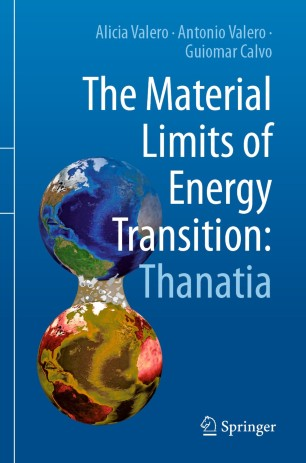Personal tools

Welcome to the Exergoecology Portal
You are here: Home / Relative Free Energy Function and Structural Theory of Thermoeconomics

# Relative Free Energy Function and Structural Theory of Thermoeconomics

This paper explores the advantages of using relative free energy instead of exergy to build a mathematical theory of thermodynamic costs to diagnose malfunctions in thermal systems. This theory is based on the definition of a linearized characteristic equation that represents the physical behavior of each component. The physical structure of the system described by its energy interrelationships is called “primal”, and its derivatives are the costs and consumptions. The obtained costing structure is the mathematical “dual” of its primal. The theory explains why the F and P cost assessment rules and any other suggestion may (or may not be) rational under a given disaggregation scheme. A result of the theory is a new thermodynamic function, called the relative free energy, and a new parameter called deterioration temperature due to a component’s deterioration cause, characterized by a h-s thermodynamic trajectory describing the effects on the exiting stream. The relative free energy function allows for an exact relationship between the amount of used resources and the increase in entropy generation caused by the deterioration path of the component. This function allows the obtaining of, for the first time, an appropriate characteristic equation for a turbine and a new definition of efficiency that does not depend on the environment temperature but on its deterioration temperature. Also, costing with relative free energy instead of exergy may open a new path for more precise and straightforward assessments of component deteriorations.

Antonio Valero Capilla and César Torres Cuadra

2020

This paper explores the advantages of using relative free energy instead of exergy to build a mathematical theory of thermodynamic costs to diagnose malfunctions in thermal systems. This theory is based on the definition of a linearized characteristic equation that represents the physical behavior of each component. The physical structure of the system described by its energy interrelationships is called “primal”, and its derivatives are the costs and consumptions. The obtained costing structure is the mathematical “dual” of its primal. The theory explains why the F and P cost assessment rules and any other suggestion may (or may not be) rational under a given disaggregation scheme. A result of the theory is a new thermodynamic function, called the relative free energy, and a new parameter called deterioration temperature due to a component’s deterioration cause, characterized by a h-s thermodynamic trajectory describing the effects on the exiting stream. The relative free energy function allows for an exact relationship between the amount of used resources and the increase in entropy generation caused by the deterioration path of the component. This function allows the obtaining of, for the first time, an appropriate characteristic equation for a turbine and a new definition of efficiency that does not depend on the environment temperature but on its deterioration temperature. Also, costing with relative free energy instead of exergy may open a new path for more precise and straightforward assessments of component deteriorations.

https://www.mdpi.com/1996-1073/13/8/2024

Energies

13

8

2024

DOI http://dx.doi.org/10.3390/en13082024

• Structural Theory
• Relative Free Energy
• Thermoeconomic analysis
• Exergy Cost Theory

April

Document Actions

unsubscribe
EXERGY MANIFESTO
RELEVANT BOOKS

## The Material Limits of Energy Transition: Thanatia

### Antonio Valero Capilla, Alicia Valero Delgado and Guiomar Calvo

This book provides a holistic view of raw mineral depletion in the context of renewable energy transistion.## mites minerales del planeta

### Antonio Valero Capilla and Alicia Valero Delgado interviewed by Adrián Almazán

We need a material transition, not only energetic, that restores nature and effectively reuses materials. Gaia must be cared for by extending life on Earth and slowing its degradation towards Thanatia.## Thermodynamics for Sustainable Management of Natural Resources### Wojciech Stanek (Editor)

This book examines ways of assessing the rational management of nonrenewable resources. Integrating numerous methods, it systematically exposes the strengths of exergy analysis in resources management.

## Thanatia: The Destiny of the Earth's Mineral Resources